﻿ Molecular properties
 Molecular properties

This block includes a set of heterogeneous molecular descriptors describing physico-chemical and biological properties as well as some molecular characteristics obtained by literature models. dProperties calculates 20 molecular properties.

The unsaturation count (Uc) is a simple information index for unsatured bonds defined as: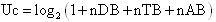where nDB, nTB and nAB are the number of of double, triple and aromatic bonds, respectively.

The unsaturation index (Ui) is calculated as: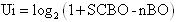where SCBO is the sum of conventional bond orders in the H-depleted molecular graph and nBO the total number of non-H bonds.

The hydrophilic factor (Hy) is a hydrophilicity descriptor defined as [R. Todeschini, M. Vighi, A. Finizio, P. Gramatica, SAR & QSAR Environ.Res. 1997, 7, 173-193]: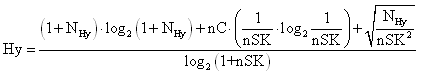where NHy is the number of hydrophilic groups (-OH, -SH, -NH), nC the number of carbon atoms and nSK the number of atoms (hydrogen excluded).

The molar refractivity (AMR) is calculated according to the Ghose-Crippen model, based on a group contribution method [A.K.Ghose and G.M.Crippen, J.Comput. Sci. 1987, 27, 21]. Each atom in every structure is classified into one of the 115 atom types. The list of the atom types with the corresponding molar refractivity contributions is given under Atom-centred fragments. The AMR estimates are provided only for compounds having atoms of types C, H, O, N, S, Se, P, B, Si, and halogens.

The topological polar surface area (TPSA) is calculated according to the model proposed by Ertl [P.Ertl et al., J.Med.Chem. 2000, 43, 3714-3717], based on a group contribution method. dProperties calculates two polar surface area descriptors, namely TPSA(NO) and TPSA(tot), the former being derived only from polar fragments with nitrogen and oxygen and the latter from polar fragments with nitrogen and oxygen plus "slightly polar" fragments containing phosphorus and sulfur.

The TPSA of a molecule is determined by the summation of tabulated surface contributions of polar atom types (see table below):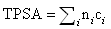where the summation runs over the defined types of polar fragments, ni is the frequency of the atom type i in the molecule, and ci is the surface contribution of atom type i. The surface contributions were calculated by least-squares fitting of the TPSA-based fragments to the single conformer 3D PSA of training set consisting of 34,810 drug-like molecules taken from the World Drug Index database. The statistical parameters of the model are: r2 = 0.982, r = 0.991, s = 7.83.

Surface contributions of polar atom types

No.

Atom type

PSA contrib.

No.

Atom type

PSA contrib.

1

[N](-*)(-*)-*

3.24

23

[nH](:*):*

15.79

2

[N](-*)=*

12.36

24

[n+](:*)(:*):*

4.10

3

[N]#*

23.79

25

[n+](-*)(:*):*

3.88

4

[N](-*)(=*)=*  (b)

11.68

26

[nH+](:*):*

14.14

5

[N](=*)#*   (c)

13.60

27

[O](-*)-*

9.23

6

[N]1(-*)-*-*-1   (d)

3.01

28

[O]1-*-*-1   (d)

12.53

7

[NH](-*)-*

12.03

29

[O]=*

17.07

8

[NH]1-*-*-1   (d)

21.94

30

[OH]-*

20.23

9

[NH]=*

23.85

31

[O-]-*

23.06

10

[NH2]-*

26.02

32

[o](:*):*

13.14

11

[N+](-*)(-*)(-*)-*

0.00

33

[S](-*)-*

25.30

12

[N+](-*)(-*)=*

3.01

34

[S]=*

32.09

13

[N+](-*)#*   (e)

4.36

35

[S](-*)(-*)=*

19.21

14

[NH+](-*)(-*)-*

4.44

36

[S](-*)(-*)(=*)=*

8.38

15

[NH+](-*)=*

13.97

37

[SH]-*

38.80

16

[NH2+](-*)-*

16.61

38

[s](:*):*

28.24

17

[NH2+]=*

25.59

39

[s](=*)(:*):*

21.70

18

[NH3+]-*

27.64

40

[P](-*)(-*)-*

13.59

19

[n](:*):*

12.89

41

[P](-*)=*

34.14

20

[n](:*)(:*):*

4.41

42

[P](-*)(-*)(-*)=*

9.81

21

[n](-*)(:*):*

4.93

43

[PH](-*)(-*)=*

23.47

22

[n](=*)(:*):*    (f)

8.39

An asterisk (*) stands for any non-hydrogen atom, - for a single bond, = for a double bond, # for a triple bond, : for an aromatic bond; atomic symbol in lowercase means that the atom is part of an aromatic system. (b) As in nitro group. (c) Middle nitrogen in azide group. (d) Atom in a three-membered ring. (e) Nitrogen in isocyano group. (f) As in pyridine N-oxide.

Moriguchi octanol-water partition coefficient (MLogP) is calculated from Moriguchi logP model consisting of a regression equation based on 13 structural parameters [I.Moriguchi, S.Hirono, Q.Liu, I.Nakagome, and Y.Matsushita, Chem.Pharm.Bull. 1992, 40, 127-130; I.Moriguchi, S.Hirono, I.Nakagome, H.Hirano, Chem.Pharm.Bull. 1994, 42, 976-978]. The regression coefficients were evaluated by a training set of 1230 organic molecules, including general aliphatic, aromatic, and heterocyclic compounds, containing the following atoms: C, H, N, O, S, P, F, Cl, Br, I. The statistical parameters of the model are: r2 = 0.906; s = 0.422.

MlogP = -1.041 + 1.244(CX)0.6 - 1.017(NO)0.9 + 0.406(PRX) - 0.145(UB)0.8 + 0.511(HB) + 0.268(POL) - 2.215(AMP) + 0.912(ALK) -0.392(RNG) -3.684(QN) + 0.474(NO2) + 1.582(NCS) + 0.773(BLM)

The model variables are frequencies (denoted by N) or presence/absence (denoted by D) of some molecular features. Their description is reported in the table below.

 Parameter Type Description CX N Summation of weighted numbers of carbon and halogen atoms; the weights are: 0.5 for F, 1.0 for C and Cl, 1.5 for Br, and 2.0 for I. NO N Total number of Ns and Os. PRX N Proximity effect of N/O: 2 for X-Y and 1 for X-A-Y (X, Y: N and/or O; A: C, S, or P; -: saturated or unsaturated bond) with a correction (-1) for -CON< and -SO2N< UB N Number of unsaturated bonds including semi-polar bonds such as N-oxides and sulfoxides, except those in NO2.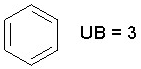HB D Dummy variable for the presence of intramolecular hydrogen bond as ortho-OH and -CO-R, -OH and -NH2, -NH2 and -COOH, or 8-OH/NH2 in quinolines, 5 or 8-OH/NH2 in quinoxalines, etc.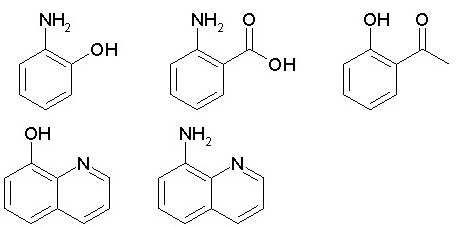POL N Number of aromatic polar substituents (aromatic substituents excluding Ar-C(X)(Y)- and Ar-C(X)=C; X, Y: C and/or H). Upper limit = 4. AMP N Amphoteric property; a-aminoacid = 1, aminobenzoic acid = 0.5, pyridinecarboxylic acid = 0.5. ALK D Dummy variable for alkane, alkene, cycloalkane, cycloalkene (hydrocarbons with 0 or 1 double bond) or hydrocarbon chain with at least 7 carbon atoms. RNG D Dummy variable for the presence of ring structures except benzene and its condensed rings (aromatic, heteroaromatic, and hydrocarbon rings).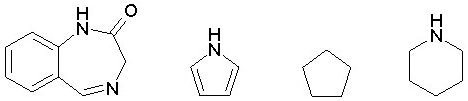QN N Quaternary nitrogen >N+<: 1; N-oxide: 0.5. NO2 N Number of nitro groups. NCS N Isothiocyanate (-N=C=S): 1.0; thiocyanate (-S-C#N): 0.5. BLM D Dummy variable for the presence of ß-lactam.

The MlogP model implemented in dProperties has been evaluated on a set of 3,576 compounds with known experimental logP taken from the NCI Open DataBase. Determination coefficient r2 resulted equal to 0.935. Moreover, on our internal logP data set comprised of 10,068 compounds the correlation coefficient r between experimental and calculated logP was 0.898.

Ghose-Crippen-Viswanadhan octanol-water partition coefficient (ALogP) is calculated from the AlogP model consisting of a regression equation based on the hydrophobicity contribution of 115 atom types [A.K. Ghose and G.M. Crippen, J. Comput. Chem. 1986, 7, 565-577; V.N. Viswanadhan et al., J. Comput. Chem. 1993, 14, 1019-1026; A.K. Ghose, V.N. Viswanadhan, J.J. Wendoloski, J.Phys.Chem. A 1998, 102, 3762-3772]. Note that AlogP estimates are provided only for compounds having atoms of types C, H, O, N, S, Se, P, B, Si, and halogens.

Each atom in every structure is classified into one of the 115 atom types. Then, estimated logP for any compound is given by: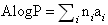where ni is the number of atom of type i and ai is the corresponding hydrophobicity constant. The list of the atom types with the corresponding hydrophobicity contributions is given under the list of atom-centred fragments.

The model coefficients are taken from Ghose et al, J.Phys.Chem. A 1998, 102, 3762-3772. They were estimated on the basis of a training set of 8364 compounds. The statistical parameters of the AlogP model are: r = 0.95; s = 0.55; predictive r2 = 0.90. The AlogP model implemented in dProperties was evaluated by the aid of a set of 3568 compounds with known experimental logP taken from the NCI Open DataBase. The resulted determination coefficient r2 was 0.931. Moreover, on our internal logP data set comprised of 9834 compounds the correlation coefficient r between experimental and calculated logP was 0.932.

Surface areas are calculated by adding atomic surface areas on the basis of the formula implemented by P_VSA-like descriptors.

McGowan volume is calculated by a group contribution method as follows: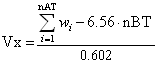where wi are Mc Gowan’s volume atomic parameters [Y.H.Zhao et al., J. Chem. Inf. Comput. Sci. 2003, 43, 1848-1854], the summation runs over the total number of atoms and nBT is the total number of bonds [M.H.Abraham and J.C.McGowan, Chromatographia 1987, 23, 243-246].

The van der Waals volume from McGowan volume (VvdwMG) is calculated as the following: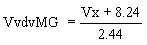where Vx is the McGowan volume.

The van der Waals volume from Zhao-Abraham-Zissimos equation (VvdwZAZ) [Y.H.Zhao et al., J. Chem. Inf. Comput. Sci. 2003, 43, 1848-1854] is calculated as the following: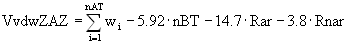where wi are the Bondi volume atom contributions, nBT the number of bonds, Rar and Rnar the number of aromatic rings and not-aromatic rings, respectively.

The packing density index (PDI) is defined as the ratio between the McGowan volume (Vx) and the total surface area from P_VSA-like descriptors (SAtot).

Three Verhaar base-line toxicities for Fish, Daphnia and Algae, respectively, are also provided as defined by Verhaar and based on Moriguchi LogP [H.J.M.Verhaar et al., Chemosphere 1992, 25, 471-491]:

BLTF96 = -0.85 * MLogP – 1.39    (Verhaar Fish)

BLTD48 = -0.95 * MLogP – 1.32    (Verhaar Daphnia)

BLTA96 = -1.00 * MLogP – 1.23     (Verhaar Algae)

For these functions, a correction factor has been introduced for logP values smaller than –6, defined as:

MLogP (corrected) = abs(MLogP) – 6